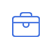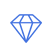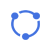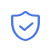# 道路运输经营许可证

• 价格透明

• 安全保密

• 准时保质

• 售后无忧

• 01 选择服务
• 02 选择顾问
• 03 在线下单
• 04 完成支付
• 05 提交资料
• 06 提供服务
• 07 验收服务
• 08 交易完成### 更多服务• 在线咨询

专业顾问在线解答
• 免费热线

18126295839 微信同号
• 公众号

•佰信官方微信

• 汇率计算

### 外币兑换计算器

 兑换金额： 持有货币： 美元(USD) 人民币(CNY) —其他货币— 欧元(EUR) 港元(HKD) 日元(JPY) 加元(CAD) 韩元(KRW) 泰铢(THB) 台币(TWD) 英镑(GBP) 荷兰盾(NLG) 澳门元(MOP) 新西兰元(NZD) 新加坡元(SGD) 捷克克朗(CZK) 挪威克郎(NOK) 丹麦克郎(DKK) 德国马克(DEM) 瑞典克朗(SEK) 瑞士法郎(CHF) 印度卢比(INR) 意大利里拉(ITL) 澳大利亚元(AUD) 菲律宾比索(PHP) 比利时法郎(BEF) 俄罗斯卢布(RUB) ——— 乌干达先令(UGX) 罗马尼亚新列伊(RON) 特立尼达多巴哥元(TTD) 圣赫勒拿群岛磅(SHP) 吉尔吉斯斯坦索姆(KGS) 吉布提法郎(DJF) 不丹努扎姆(BTN) 南非兰特(ZAR) 以色列新锡克尔(ILS) 叙利亚磅(SYP) 海地古德(HTG) 也门里亚尔(YER) 乌拉圭比索(UYU) 巴巴多斯元(BBD) 盎司黄金(XAU) 爱沙尼亚克鲁恩(EEK) 芬兰马克(FIM) 马拉维克瓦查(MWK) 印尼盾(IDR) 罗马尼亚列伊(ROL) 巴布亚新几内亚基那(PGK) 斯洛文尼亚托拉尔(SIT) 格林纳达东加勒比元(XCD) 卢旺达法郎(RWF) 尼日利亚奈拉(NGN) 土库曼斯坦马纳特(TMM) 巴哈马元(BSD) 克罗地亚库纳(HRK) 哥伦比亚比索(COP) 乔治亚拉里(GEL) 瓦努阿图瓦图(VUV) 斐济元(FJD) 马尔代夫罗非亚(MVR) 阿塞拜疆曼纳特(AZN) 蒙古图格里克(MNT) 马达加斯加阿里亚里(MGA) 爱尔兰镑(IEP) 苏里南盾(SRG) 科摩罗法郎(KMF) 几内亚法郎(GNF) 所罗门元(SBD) 科威特第纳尔(KWD) 孟加拉塔卡(BDT) 委内瑞拉博利瓦(VEB) 缅元(MMK) 土耳其里拉(TRL) 塔吉克斯坦索莫尼(TJS) 约旦第纳尔(JOD) 巴拿马巴波亚(PAB) 摩尔多瓦列伊(MDL) 佛得角埃斯库多(CVE) 智利比索(CLP) 肯尼亚先令(KES) 苏里南元(SRD) 毛里求斯卢比(MUR) 利比里亚元(LRD) 沙特阿拉伯里亚尔(SAR) 阿根廷比索(ARS) 埃及镑(EGP) 巴拉圭瓜尼(PYG) 土耳其新里拉(TRY) 刚果法郎(CDF) 百慕大元(BMD) 阿曼里亚尔(OMR) 古巴比索(CUP) 尼加拉瓜科多巴(NIO) 冈比亚达拉西(GMD) 斯洛伐克克朗(SKK) 乌兹别克斯坦苏姆(UZS) 赞比亚克瓦查(ZMK) 危地马拉格查尔(GTQ) 尼泊尔卢比(NPR) 纳米比亚元(NAD) 欧元（旧）(XEU) 匈牙利福林(HUF) 老挝基普(LAK) 斯威士兰里兰吉尼(SZL) 沙特阿拉伯里亚尔(UDI) 马耳他里拉(MTL) 文莱币(BND) 坦桑尼亚先令(TZS) 苏丹镑(SDG) 莱索托洛蒂(LSL) 开曼群岛币(KYD) 斯里兰卡卢比(LKR) 马其顿第纳尔(MKD) 墨西哥比索(MXN) 加纳塞第(GHC) 冰岛克郎(ISK) 利比亚第纳尔(LYD) 塞拉里昂利昂(SLL) 巴基斯坦卢比(PKR) 安第列斯群岛盾(ANG) 塞舌尔卢比(SCR) 奥地利先令(ATS) 黎巴嫩镑(LBP) 阿联酋迪拉姆(AED) 新加纳塞第(GHS) 玻利维亚币(BOB) 厄立特里亚纳克法(ERN) 直布罗陀镑(GIP) 卡塔尔里亚尔(QAR) 巴林第纳尔(BHD) 伊朗里亚尔(IRR) 博茨瓦纳普拉(BWP) 洪都拉斯伦皮拉(HNL) 阿尔巴尼亚列克(ALL) 赛尔维亚第纳尔(RSD) 马来西亚林吉特(MYR) 埃塞俄比亚比尔(ETB) 圣多美和普林西比多布拉(STD) 安道尔西班牙银币(ADP) 保加利亚列弗(BGN) 多米尼加比索(DOP) 亚美尼亚打兰(AMD) 法国太平洋法郎(XPF) 特别提款权(SDR) 牙买加元(JMD) 毛里塔尼亚乌吉亚(MRO) 加那利群岛比塞塔(ESP) 津巴布韦元(ZWD) 拉脱维亚拉茨(LVL) 布隆迪法郎(BIF) 马提尼克法郎(FRF) 突尼斯第纳尔(TND) 厄瓜多尔苏克雷(ECS) 越南盾(VND) 希腊德拉克马(GRD) 秘鲁新索尔(PEN) 阿尔及利亚第纳尔(DZD) 莫桑比克梅蒂卡尔(MZN) 阿鲁巴弗罗林(AWG) 莫桑比克美提卡(MZM) 多哥非共体法郎(XOF) 葡萄牙埃斯库多(PTE) 哈萨克斯坦坚戈(KZT) 乌克兰赫夫米(UAH) 伯利兹元(BZD) 波斯尼亚(BAM) 摩洛哥迪拉姆(MAD) 白俄罗斯卢布(BYR) 立陶宛立特(LTL) 柬埔寨瑞尔(KHR) 西非法郎(XAF) 塞浦路斯镑(CYP) 圭亚那元(GYD) 巴西雷亚尔(BRL) 阿富汗尼(AFN) 哥斯达黎加科朗(CRC) 萨尔瓦多科朗(SVC) 伊拉克第纳尔(IQD) 波兰兹罗提(PLN) 索马里先令(SOS) 汤加潘加(TOP) 安哥拉宽扎(AOA) 卢森堡法郎(LUF) 朝鲜元(KPW) 兑换货币： 人民币(CNY) 美元(USD) —其他货币— 欧元(EUR) 港元(HKD) 日元(JPY) 加元(CAD) 韩元(KRW) 泰铢(THB) 台币(TWD) 英镑(GBP) 荷兰盾(NLG) 澳门元(MOP) 新西兰元(NZD) 新加坡元(SGD) 捷克克朗(CZK) 挪威克郎(NOK) 丹麦克郎(DKK) 德国马克(DEM) 瑞典克朗(SEK) 瑞士法郎(CHF) 印度卢比(INR) 意大利里拉(ITL) 澳大利亚元(AUD) 菲律宾比索(PHP) 比利时法郎(BEF) 俄罗斯卢布(RUB) ——— 乌干达先令(UGX) 罗马尼亚新列伊(RON) 特立尼达多巴哥元(TTD) 圣赫勒拿群岛磅(SHP) 吉尔吉斯斯坦索姆(KGS) 吉布提法郎(DJF) 不丹努扎姆(BTN) 南非兰特(ZAR) 以色列新锡克尔(ILS) 叙利亚磅(SYP) 海地古德(HTG) 也门里亚尔(YER) 乌拉圭比索(UYU) 巴巴多斯元(BBD) 盎司黄金(XAU) 爱沙尼亚克鲁恩(EEK) 芬兰马克(FIM) 马拉维克瓦查(MWK) 印尼盾(IDR) 罗马尼亚列伊(ROL) 巴布亚新几内亚基那(PGK) 斯洛文尼亚托拉尔(SIT) 格林纳达东加勒比元(XCD) 卢旺达法郎(RWF) 尼日利亚奈拉(NGN) 土库曼斯坦马纳特(TMM) 巴哈马元(BSD) 克罗地亚库纳(HRK) 哥伦比亚比索(COP) 乔治亚拉里(GEL) 瓦努阿图瓦图(VUV) 斐济元(FJD) 马尔代夫罗非亚(MVR) 阿塞拜疆曼纳特(AZN) 蒙古图格里克(MNT) 马达加斯加阿里亚里(MGA) 爱尔兰镑(IEP) 苏里南盾(SRG) 科摩罗法郎(KMF) 几内亚法郎(GNF) 所罗门元(SBD) 科威特第纳尔(KWD) 孟加拉塔卡(BDT) 委内瑞拉博利瓦(VEB) 缅元(MMK) 土耳其里拉(TRL) 塔吉克斯坦索莫尼(TJS) 约旦第纳尔(JOD) 巴拿马巴波亚(PAB) 摩尔多瓦列伊(MDL) 佛得角埃斯库多(CVE) 智利比索(CLP) 肯尼亚先令(KES) 苏里南元(SRD) 毛里求斯卢比(MUR) 利比里亚元(LRD) 沙特阿拉伯里亚尔(SAR) 阿根廷比索(ARS) 埃及镑(EGP) 巴拉圭瓜尼(PYG) 土耳其新里拉(TRY) 刚果法郎(CDF) 百慕大元(BMD) 阿曼里亚尔(OMR) 古巴比索(CUP) 尼加拉瓜科多巴(NIO) 冈比亚达拉西(GMD) 斯洛伐克克朗(SKK) 乌兹别克斯坦苏姆(UZS) 赞比亚克瓦查(ZMK) 危地马拉格查尔(GTQ) 尼泊尔卢比(NPR) 纳米比亚元(NAD) 欧元（旧）(XEU) 匈牙利福林(HUF) 老挝基普(LAK) 斯威士兰里兰吉尼(SZL) 沙特阿拉伯里亚尔(UDI) 马耳他里拉(MTL) 文莱币(BND) 坦桑尼亚先令(TZS) 苏丹镑(SDG) 莱索托洛蒂(LSL) 开曼群岛币(KYD) 斯里兰卡卢比(LKR) 马其顿第纳尔(MKD) 墨西哥比索(MXN) 加纳塞第(GHC) 冰岛克郎(ISK) 利比亚第纳尔(LYD) 塞拉里昂利昂(SLL) 巴基斯坦卢比(PKR) 安第列斯群岛盾(ANG) 塞舌尔卢比(SCR) 奥地利先令(ATS) 黎巴嫩镑(LBP) 阿联酋迪拉姆(AED) 新加纳塞第(GHS) 玻利维亚币(BOB) 厄立特里亚纳克法(ERN) 直布罗陀镑(GIP) 卡塔尔里亚尔(QAR) 巴林第纳尔(BHD) 伊朗里亚尔(IRR) 博茨瓦纳普拉(BWP) 洪都拉斯伦皮拉(HNL) 阿尔巴尼亚列克(ALL) 赛尔维亚第纳尔(RSD) 马来西亚林吉特(MYR) 埃塞俄比亚比尔(ETB) 圣多美和普林西比多布拉(STD) 安道尔西班牙银币(ADP) 保加利亚列弗(BGN) 多米尼加比索(DOP) 亚美尼亚打兰(AMD) 法国太平洋法郎(XPF) 特别提款权(SDR) 牙买加元(JMD) 毛里塔尼亚乌吉亚(MRO) 加那利群岛比塞塔(ESP) 津巴布韦元(ZWD) 拉脱维亚拉茨(LVL) 布隆迪法郎(BIF) 马提尼克法郎(FRF) 突尼斯第纳尔(TND) 厄瓜多尔苏克雷(ECS) 越南盾(VND) 希腊德拉克马(GRD) 秘鲁新索尔(PEN) 阿尔及利亚第纳尔(DZD) 莫桑比克梅蒂卡尔(MZN) 阿鲁巴弗罗林(AWG) 莫桑比克美提卡(MZM) 多哥非共体法郎(XOF) 葡萄牙埃斯库多(PTE) 哈萨克斯坦坚戈(KZT) 乌克兰赫夫米(UAH) 伯利兹元(BZD) 波斯尼亚(BAM) 摩洛哥迪拉姆(MAD) 白俄罗斯卢布(BYR) 立陶宛立特(LTL) 柬埔寨瑞尔(KHR) 西非法郎(XAF) 塞浦路斯镑(CYP) 圭亚那元(GYD) 巴西雷亚尔(BRL) 阿富汗尼(AFN) 哥斯达黎加科朗(CRC) 萨尔瓦多科朗(SVC) 伊拉克第纳尔(IQD) 波兰兹罗提(PLN) 索马里先令(SOS) 汤加潘加(TOP) 安哥拉宽扎(AOA) 卢森堡法郎(LUF) 朝鲜元(KPW) 兑换结果：
• 返回顶部